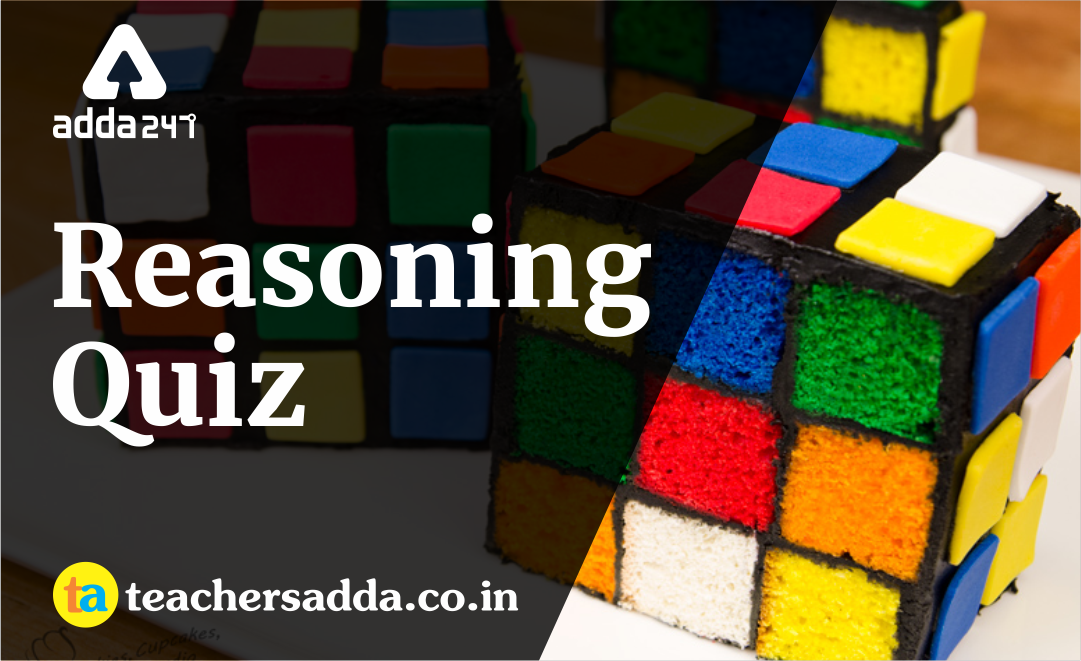The reasoning is the most scoring section in any competitive exam. It also develops or nourishes our critical thinking skills as compared to other subjects. In DSSSBKVSAPS Exam as a student to score well in this part, you have to start your preparation now because there is negative marking as well. The more you have prepared, the better you score.

Q1. Excluding stoppages, the speed of a bus is 54 kmph and including stoppages, it is 45kmph. For how many minutes does the bus stop per hour?
(a) 9
(b) 10
(c) 12
(d) 20

Q2. Pointing towards A, B said “your mother is the younger sister of my mother”. How is A related to B?
(a) Uncle
(b) Cousin
(c) Nephew
(d) Father

Directions (Q3): In each of the following questions, from the given alternatives select the word which cannot be formed using the letters of the given word.
Q3. FRAMEWORK
(a) MARK
(b) FAME
(c) FOUR
(d) MORE

Q4. If train is called bus, bus is called tractor, tractor is called car, car is called scooter, scooter is called bicycle, bicycle is called moped, which is used to plough a field?
(a) Train
(b) Bus
(c) Tractor
(d) Car

Q5. If 7+5=144  and 3+2=25,then 9+7= ?
(a) 49
(b) 256
(c) 81
(d) 121

Directions (Q6-7): In each of the following questions, select the missing number from the given responses.(a) 13
(b) 11
(c) 14
(d)  9(a) 8
(b) 16
(c) 64
(d) 4

Q8. John and Khan start together from a particular point in the opposite direction on motorcycle.  The speed of John is 25 km/hr and Khan is 35 km/hr. What will be the distance between them after 15 minutes?
(a) 15 km
(b) 20 km
(c) 5 km
(d) 10 km(a)
(b)
(c)
(d)

Q10.Triangle represents people in the first age group i.e. 40-50 years, Square represents the second age group i. e. 60-70 years and Rectangle represents the third age group i. e. 20-30 years. The portion which represents all the three age groups is
(a) 2
(b) 7
(c) 4
(d) 6

Solutions

S1. Ans.(b)S2. Ans.(b)
Sol. The mothers of A and B are sisters. Therefore, A and B are cousins.

S3. Ans.(c)
Sol. There is no ‘U’ letter in the given word. Therefore, the word FOUR cannot be formed.

S4. Ans.(d)
Sol. Tractor is used to plough field. But, here tractor has been balled ‘car’.

S5. Ans.(b)S6. Ans.(d)S7. Ans.(d)
Sol.S8. Ans.(a)
Sol. Relative speed of John and Khan
=(25+35)=60 kmph
Distance covered in 15 minutes
=60×15/60=15 km

S9. Ans.(c)
Sol.S10. Ans.(d)
Sol. The number ‘6’ is common to all three geometrical figures.